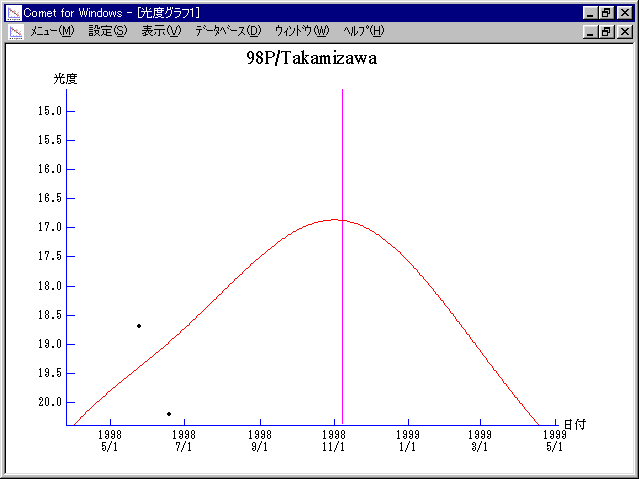# \$B9b8+BtWB@1(B

98P/Takamizawa (1998)###\$B50F;MWAG(B

```   The following improved orbital elements by Kenji Muraoka,
are from 151 observations 1984 to 1998, including 9 Planets,
Moon and 5 minor planets perturbations and non-gravitational
effect of style II.  The mean residual is +/- 0.89 arc seconds.

Epoch  =  1998 Nov.  3.0  TT       JDT = 2451120.5
T  =  1998 Nov.  7.99422       +/- 0.00091 (m.e.) TT
Peri. =  147.80194                +/- 0.00056
Node  =  124.84585                +/- 0.00009   (2000.0)
Incl. =    9.48975                +/- 0.00002
q  =    1.5852200              +/- 0.0000021 AU
e  =    0.5751663              +/- 0.0000018
a  =    3.7313894              +/- 0.0000043 AU
n  =    0.13674083             +/- 0.00000024
P  =    7.208                  +/- 0.0000126  years
A1  =   +0.691                  +/- 0.158
A2  =   +0.02575                +/- 0.00214
```

###\$B@1?^(B###\$B8wEYJQ2=(B

```        m1 = 11.0 + 5 log\$B&\$(B + 20 log r
```##### \$B50F;MWAG\$OB<2,7r<#;a\$N7W;;\$K\$h\$k\$b\$N\$G\$9!#(B \$B@1?^\$O(B StellaNavigator Ver.2.0 for Windows (\$B%"%9%H%m%"!<%D(B \$BJTCx(B / \$B%"%9%-!<=PHG6I4)(B) \$B\$G:n@.\$7\$?\$b\$N\$G\$9!#(B \$B8wEY%0%i%U\$O(BComet for Windows\$B\$G:n@.\$7\$?\$b\$N\$G\$9!#(B